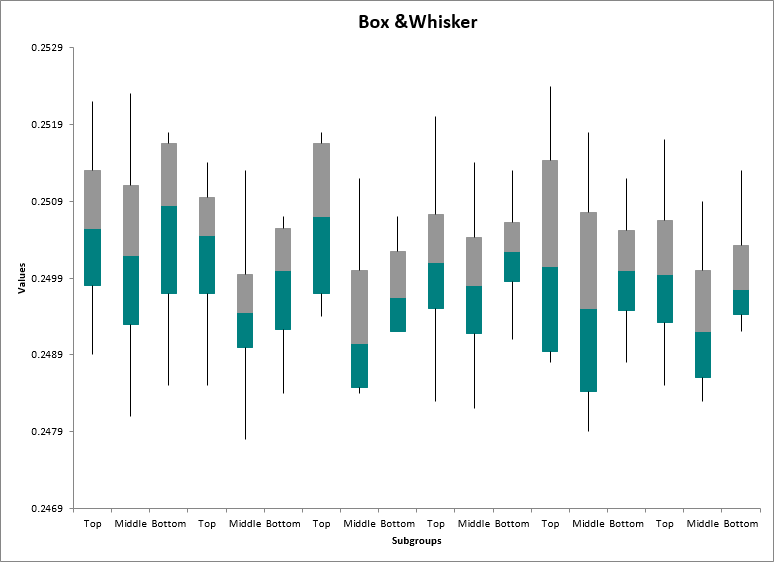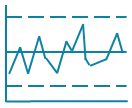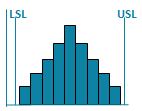# Values Plot in Excel

## Use a Values Plot to show the spread of individual data points.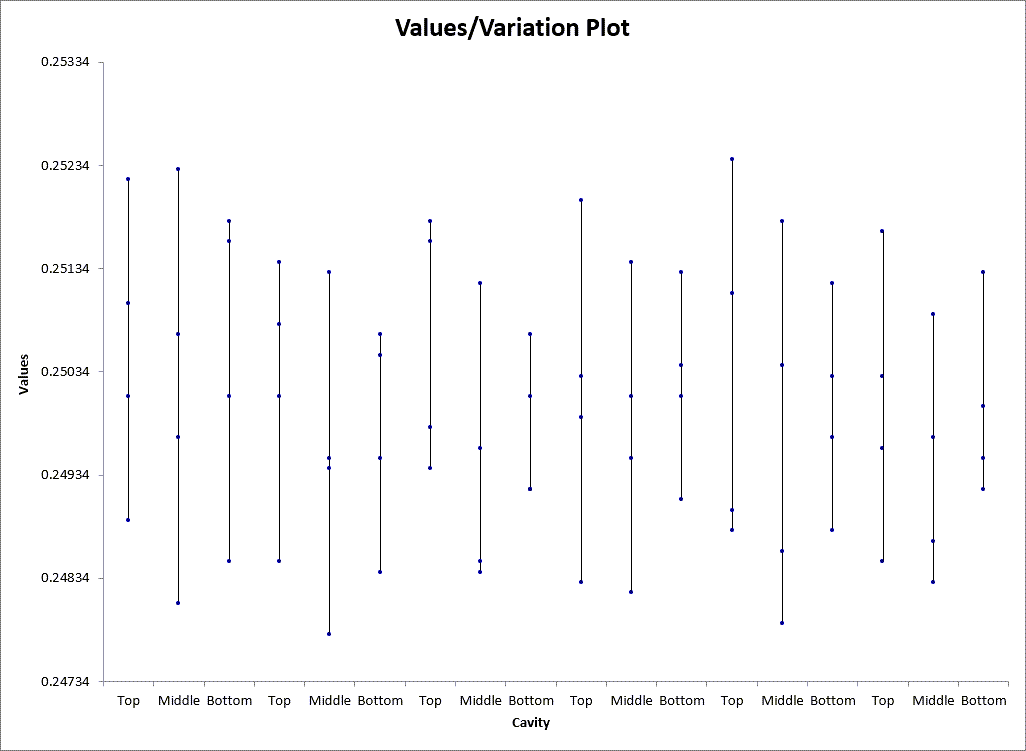### How to Create a Values Plot in Excel

While its possible to create a values plot in Excel, it is very time consuming and you have to know what all of the steps are. To save time, consider creating a values plot using QI Macros add-in for Excel. Its super simple and takes just seconds.

QI Macros installs a new tab on Excel's menu.

1. First click and drag over your data to select it.
2. Then click on QI Macros menu and select Box, Dot,& Scatter Plot and then Values Plot: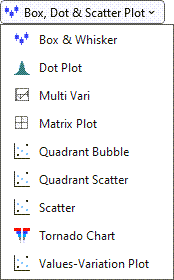3. QI Macros will perform all of the calculations and draw a values plot for you.

### Compare a Values Plot to a Box and Whisker Plot

Using the same data, you can also run a Box and Whisker Plot which will perform a more robust analysis of your data. QI Macros can create box and whisker plots too.

#### Values Plot#### Box and Whisker Plot